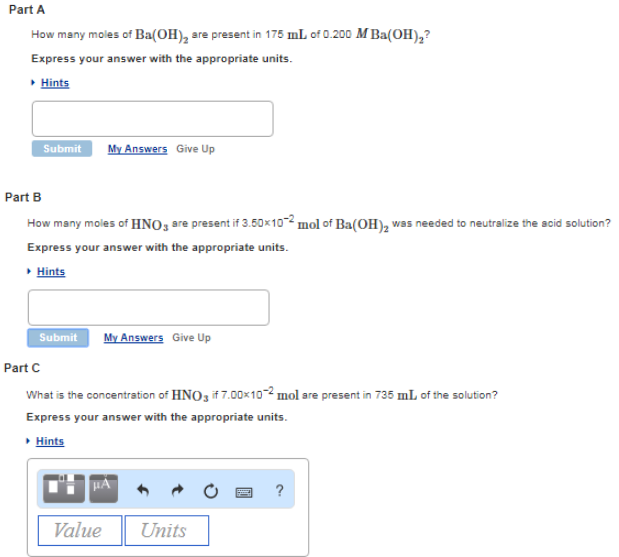# Problem: Part AHow many moles of Ba(OH)2 are present in 175 mL of 0.200 M Ba(OH)2? Express your answer with the appropriate units. Part BHow many moles of HNO3 are present if 3.50 x 10-2 mol of Ba(OH)2 was needed to neutralize the acid solution? Express your answer with the appropriate units. Part CWhat is the concentration of HNO3 if 7.00 x 10-2 mol are present in 735 mL of the solution? Express your answer with the appropriate units.

###### FREE Expert Solution
91% (55 ratings)###### Problem Details

Part A

How many moles of Ba(OH)2 are present in 175 mL of 0.200 M Ba(OH)2? Express your answer with the appropriate units.

Part B

How many moles of HNO3 are present if 3.50 x 10-2 mol of Ba(OH)2 was needed to neutralize the acid solution? Express your answer with the appropriate units.

Part C

What is the concentration of HNO3 if 7.00 x 10-2 mol are present in 735 mL of the solution? Express your answer with the appropriate units.